# Routing with GRASS GIS: Catchment Area Calculation

I got my hands on pgRouting in the last post and I’m about to do the same with GRASS GIS in this one.

GRASS GIS stores the topology for the native vector format by default, which makes it easy to use for the network analysis. All the commands associated with the network analysis can be found in the `v.net` family. The ones I’m going to discuss in this post are `v.net` itself, `v.net.path`, `.v.net.alloc` and `v.net.iso`, respectively.

## Data

I’m going to use the roads data from the previous post together with some random points used as catchment areas centers.

```# create the new GRASS GIS location
grass -text -c ./osm/czech

# import the random points
v.in.ogr input="PG:host=localhost dbname=pgrouting" layer=temp.points output=points -eo --overwrite
```

I got six different points and the pretty dense road network. Note none of the points is connected to the existing network.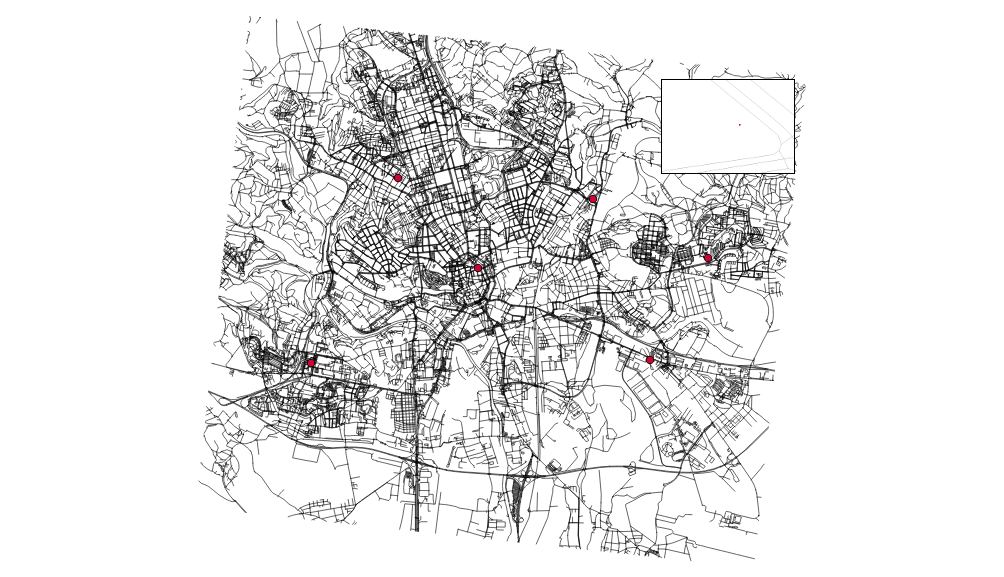You have to have routable network to do the actual routing (the worst sentence ever written). To do so, let’s:

• connect the random points to the network

Note I’m using the 500m as the max distance in which to connect the points to the network.

```v.net input=roads points=points operation=connect threshold=500 output=network
v.net input=network output=network_noded operation=nodes
```

## Finding the shortest path between two points

Once the network is routable, it is easy to find the shortest path between points number 1 and 4 and store it in the new map.

```echo "1 1 4" | v.net.path input=network_noded output=path_1_4
```

The algorithm doesn’t take bridges, tunnels and oneways into account, it’s capable of doing so though.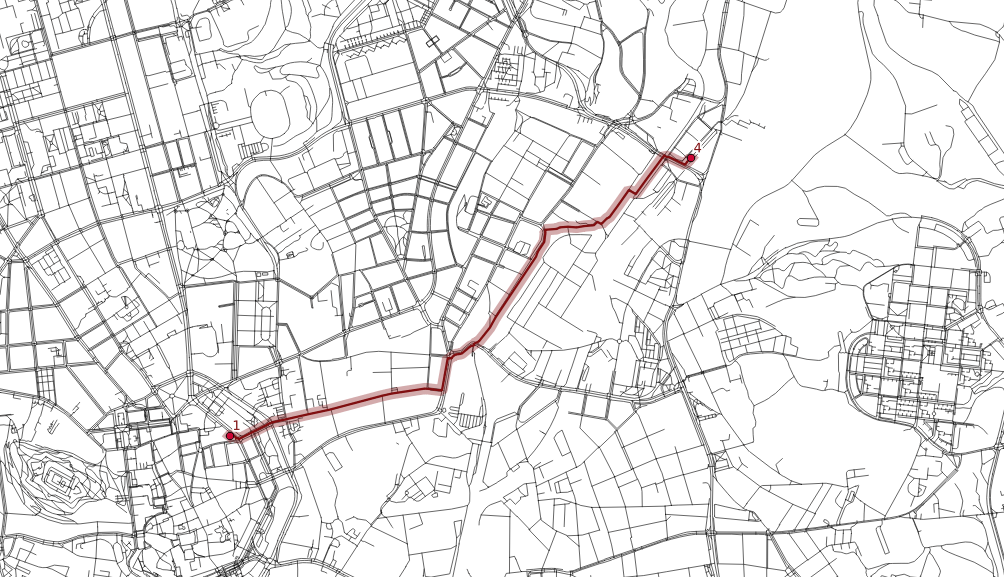## Distributing the subnets for nearest centers

```v.net.alloc input=network_noded output=network_alloc center_cats=1-6 node_layer=2
```

`v.net.alloc` module takes the given centers and distributes the network so each of its parts belongs to exactly one center - the nearest one (speaking the distance, time units, …).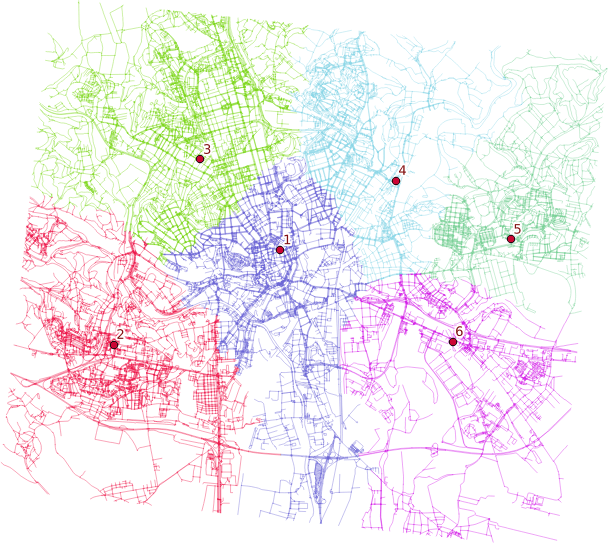## Creating catchment areas

```v.net.iso input=network_noded output=network_iso center_cats=1-6 costs=1000,3000,5000
```

`v.net.iso` splits net by cost isolines. Again, the costs might be specified as lengths, time units, ….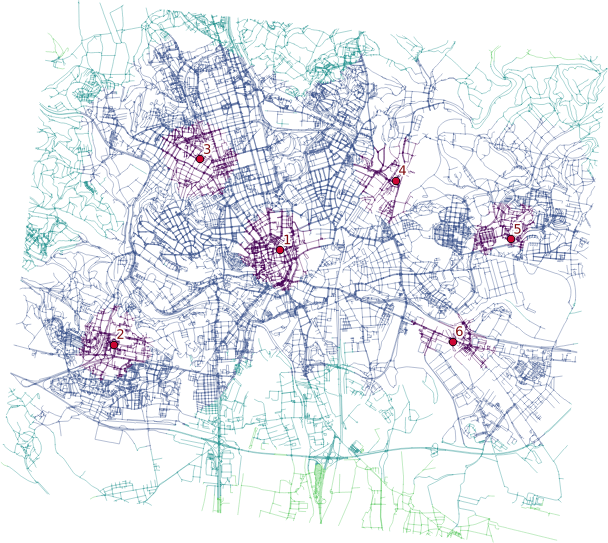Two different ways lead to the actual catchment area creation. First, you extract nodes from the roads with their values, turn them into the raster grid and either extract contours or polygonize the raster. I find the last step suboptimal and would love to find another way of polygonizing the results.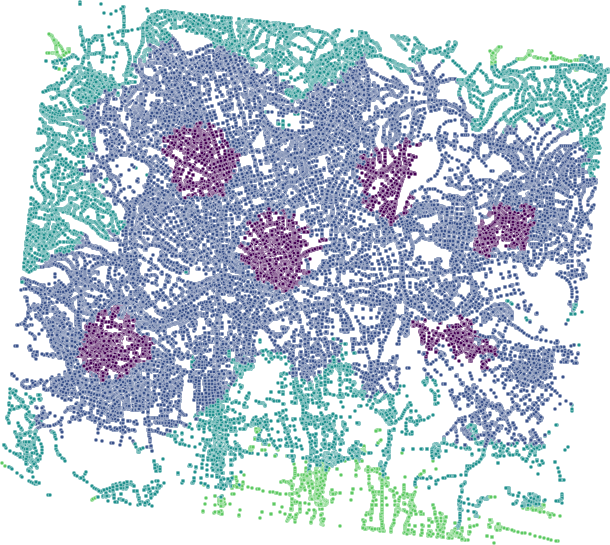Note when extracting contours the interval has to be set to the reasonable number depending on the nodes values.

## Remarks

• Once you grasp the basics, GRASS GIS is real fun. Grasping the basics is pretty tough though.
• Bridges and tunnels might be an issue.
• Personally, I find GRASS GIS easier to use for the network analysis compared to pgRouting.

# GRASS: Big Buffers Made Easy

Recently I’ve written about struggling with fairly complex geometries in PostGIS. Lesson learned from the last time was to use more smaller geometries instead of several really huge. You can obtain the small ones from the big by cutting it with a grid.

A supervisor of a project I’ve been working on came up with a task that totally buried the previous process in a blink of an eye: Give me the buffer (diffed with original geometries) that is smoothed on the outer bounds so there are no edges shorter than 10 cm. I sighed. Then, I cursed. Then, I gave it a try with PostGIS. Achieving this goal involves these steps:

• Dissolve intersecting buffers
• Run some kind of generalization algorithm that is not defined in PostGIS
• Diff original geometries
• Cut buffer with grid so it is faster not so slow for the next steps

Two of those four are rather problematic with PostGIS: line smoothing and diffing the original geometries (I didn’t get to the last one, so it might be 3 of 4 as well).

## Hello, I’m GRASS

I haven’t used GRASS for ages and even back then I didn’t get to know it much, but it saved the day for me this time.

```grass -text path/to/mapset -c

v.in.ogr input="PG:host=localhost dbname=db user=postgres password=postgres" output=ilot_050 layer=ilot_2015_050 snap=-1 --overwrite
# turn the snapping off, I don't want the input changed in any way, even though it is not topologically valid
v.in.ogr input="PG:host=localhost dbname=db user=postgres password=postgres" output=lollipops_050 layer=lollipops.lollipops_2015_050_tmp snap=-1 --overwrite
v.in.ogr input="PG:host=localhost dbname=db user=postgres password=postgres" output=holes_050 layer=phase_3.holes_050 snap=-1 --overwrite
v.db.update map=ilot_050 column=id_0 value=1

# dissolve didn't work without a column specified, dunno why
v.dissolve input=ilot_050 column=id_0 output=ilot_050_dissolve --overwrite
v.buffer input=ilot_050_dissolve output=ilot_050_buffer distance=20 --overwrite

# v.out and v.in routine used just because I didn't get the way attributes work in GRASS, would do it differently next time
v.out.ogr input=ilot_050_buffer output=ilot_050_buffer format=ESRI_Shapefile --overwrite
v.in.ogr input=ilot_050_buffer output=ilot_050_buffer snap=-1 --overwrite
v.overlay ainput=ilot_050_buffer binput=holes_050 operator=or output=combined_050_01 snap=-1 --overwrite

# tried v.patch to combine the three layers, it gave some strange results in the final overlay
v.overlay ainput=combined_050_01 binput=lollipops_050 operator=or output=combined_050_02 snap=-1 --overwrite
v.out.ogr input=combined_050_02 output=combined_050 format=ESRI_Shapefile --overwrite
v.in.ogr input=combined_050 output=combined_050_in snap=-1 --overwrite
v.db.update map=combined_050_in column=id_1 value=1
v.dissolve input=combined_050_in column=id_1 output=combined_050_dissolve --overwrite

# get rid of &lt; 10cm edges
v.generalize input=combined_050_dissolve output=combined_050_gen method=reduction threshold=0.1 --overwrite
v.out.ogr input=combined_050_gen output=combined_050_gen format=ESRI_Shapefile --overwrite
v.in.ogr input=combined_050_gen output=combined_050_gen snap=-1 --overwrite
v.overlay ainput=combined_050_gen binput=ilot_050 operator=not snap=1e-05 --overwrite output=ilot_050_diff
v.out.postgis input=ilot_050_diff output="PG:dbname=db user=postgres password=postgres" output_layer=onf3.buffer_050_diff options="GEOMETRY_NAME=wkb_geometry,SRID=2154" --overwrite
v.in.ogr input="PG:host=localhost dbname=ign user=postgres password=postgres" output=buffer_050 layer=onf3.buffer_050_diff snap=-1 --overwrite
v.in.ogr input="PG:host=localhost dbname=ign user=postgres password=postgres" output=grid layer=grid snap=-1 --overwrite
v.db.connect -d map=buffer_050

# instead of v.out and v.in routine
db.connect driver=sqlite database='\$GISDBASE/\$LOCATION_NAME/\$MAPSET/sqlite.db'
v.overlay ainput=buffer_050 binput=grid operator=and output=buffer_050_grid snap=-1 --overwrite
v.out.postgis input=buffer_050_grid output="PG:dbname=ign user=postgres password=postgres" output_layer=onf3.buffer_050_diff_grid options="GEOMETRY_NAME=wkb_geometry,SRID=2154" --overwrite
```

It is damn fast compared to PostGIS. It can be automated. It can be parametrized. It is robust. It is great!

## Lesson learned

• You cannot smooth lines by deleting edges shorter than `n` in PostGIS. At least I haven’t found the way to do so without defining your own procedure. You can with GRASS.
• GRASS reduction algorithm always keep first and last node untouched. Thus, if they’re closer than `n`, they’ll stay even if you’d like to have them deleted.
• Getting to grips with GRASS attribute data is rather hard after using shapefiles all your GIS life.
• It is great to exploit synergy of different GIS tools used for what they’re best at.

The more I work with big data, the more I get used to not seeing them. That’s kind of a twist after crafting maps at university.